Practice
Resources
Contests
Online IDE
New
Free Mock
Scaler
Practice
Improve your coding skills with our resources
Contests
Compete in popular contests with top coders
Scaler
Explore Offerings by SCALER## Welcome to Interviewbit, help us create the best experience for you!

Currently, You are a:College/University *
Enter the name of your college
Branch *
Year of completion *College/University *
Enter the name of your college
Branch *
Year of completion *Current Company *
Enter company name
Experience *## You're all set!Full name *
Email *

By creating an account, I acknowledge that I have read and agree to InterviewBit’s Terms and Privacy Policy .## Welcome back!

Email *Go to Problems

Be a Code Ninja!

# Binary Search Tree

Binary Search Tree is just another binary tree with the twist where the scanned input goes either to the left or to the right of the root node also called as the parent node. All elements to the left are the ones that are lesser than the value at the root node. And all elements to the right are the ones greater than the value at the root node. The values to the left and right are called as the children to the root (parent). And their children are collectively called as sub-trees.

The basic purpose and idea behind such a data structure are to have a storing mechanism that provides a way for efficient sorting, searching, traversing, insertion and deletion operations.

## Working of Binary Search Tree:

1. The Binary search tree works in a manner where every element that is to be inserted gets sorted then and there itself upon insertion.
2. The comparison starts with the root, thereby following the left or right sub-tree depending if the value to be inserted is lesser or greater than root, respectively.
3. Thus any value which is to be inserted is compared with the value at the root. Any value less than the root gets inserted in the left child and its children, whereas any value greater than the root gets inserted in the right child and its children.
4. Thus all the values get automatically sorted as they’re inserted into the tree.

## Implementation:

Let us understand the basic operations in a binary search tree:

1. Insertion

Case 1: Root of the tree is present, with no children.

When the root is present and has no children, then the next element to be inserted is compared with the value at the root node.

If the value to be inserted is greater than the value at root, it is inserted as the right child of the root.

If the value to be inserted is smaller than the value at root, it is inserted as the left child of the root.

Case 2: When no node is present, i.e., the tree gets its first node.

When there isn’t any node in the tree or the situation where the first node is to be inserted. No matter what the value is, the first element becomes the root node. All other nodes that are to be inserted after the first node, the insertion takes place according to Case 1.

Case 3: When root and its children are already present.

When the root and its children are already present, the next element to be inserted either gets inserted in the left sub-tree or right sub-tree. First, the element is compared with the value at the root node.

If the value is greater than the value at the root node, we proceed to the right sub-tree and follow the same approach of comparing the value with the first right child, followed by its left and right child.

If the value is smaller than the value at the root node, we proceed to the left sub-tree and follow the same approach of comparing the value of the first left child, followed by its left and right child.

This is done until one finds the right position for the value-to-be-inserted.

An example might come in handy for understanding the concept.

Insert the following elements to form a binary search tree: 11, 2, 9, 13, 57, 25, 1, 1, 90, 3. The first number to be inserted is 11. Since there are no nodes present thus 11 becomes the root node.The next element to be inserted is 2.

We first compare 2 with 11. Since 2 is less than 11. 2 will become the left child of 11.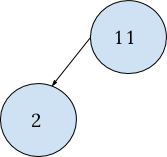The next element to be inserted is 9. Compare 9 with 11. Since 9 is less than 11, it will proceed towards the left sub-tree of 11 until it finds its final position.

Now we compare 9 with the first element of the left sub-tree of 11.

The first element of the left sub-tree is 2. We compare 9 and 2. Since 9 is greater than 2, it will be inserted as the right child of 2.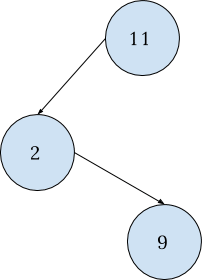Next in line is 13. We compare 13 with 11. Since 13 is greater than 11, thus it will be inserted in the right sub-tree of 11. But there’s no element present to the right of 11, thus 13 becomes the right child of 11.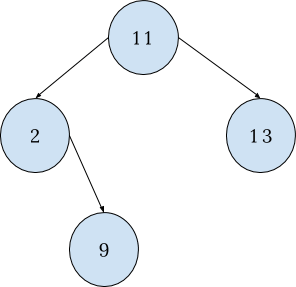Follow the same approach for the remaining elements till your final tree looks like this: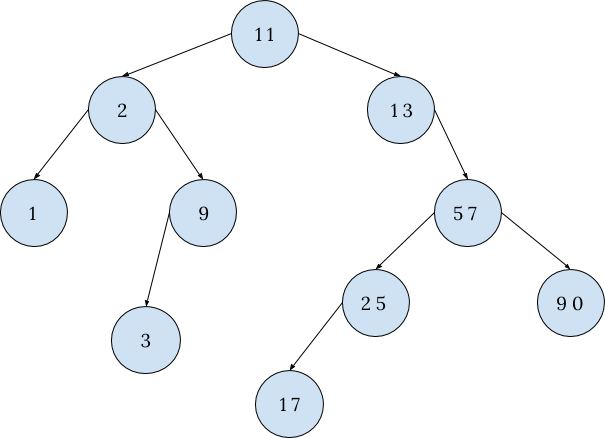1. Deletion

Deletion of any element from the binary search tree or any tree for that matter is a tricky business. The cases to consider for the element to be deleted are as follows:

Case 1: The element to be deleted is a leaf node. (A node with no children)

The removal or deletion of a leaf node does not affect the tree and its other functions as it neither has children nor does the parent has any effect due to the same thing. Use the search function to find the node to be deleted.

Once found, remove (delete) the node.

From the example quoted above, we delete the element 17.

17 is a leaf node, as shown in the diagram.

We search for the element first starting from the root and continuing through its children till we find the node to be deleted.

After the node is found, we can easily remove it.

The tree after deleting 17 looks like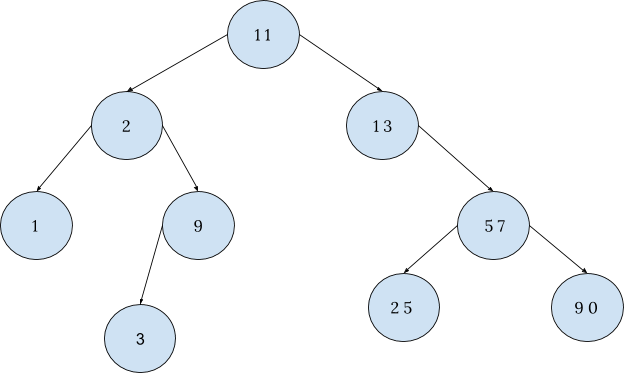Case 2: Node that is to be deleted has one child

The deletion of a node that has only one child is similar to the deletion of a node from a linked list.

The child’s connection is first made with the parent of the node to be deleted and then the node is deleted.

If we’re to delete number 13, the picture below shows how: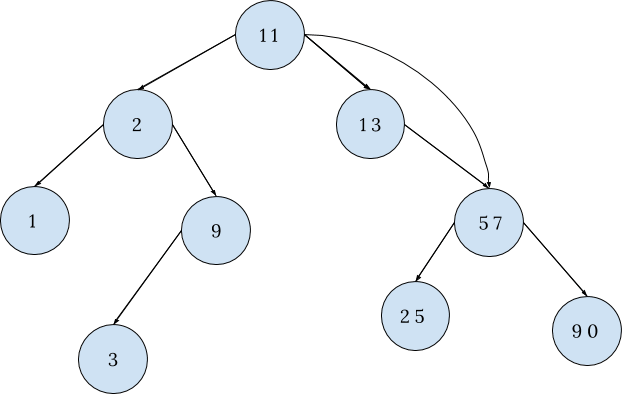After the connection is made, 13 can now be deleted. The Binary search tree after deleting 13 looks like: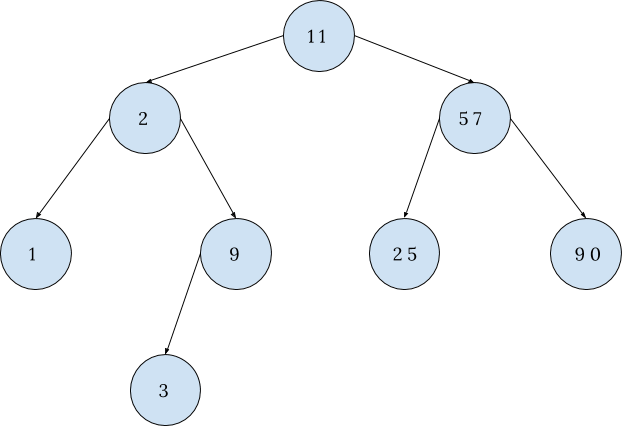Case 3: Node to be deleted has two children.

The node which is to be deleted has two children, we find the node with closest value to the value of the node to be deleted. This value may be:

1. Smallest value in the right subtree, or
2. Largest value in the left subtree (We may choose this when there is no right subtree present)

Now swap the values of the resultant node and the node to be deleted.

Look at the tree, if the structure and situation are either of the two cases, case 1 and case 2 respectively. According to the found case, proceed.

Here we wish to delete 2. Let us see how it is done.

The possible substitute would be either 1 (i.e., largest value in the left subtree), or 3 (i.e., smallest value in right subtree).

Since we have right subtree present, we may swap the node with 3.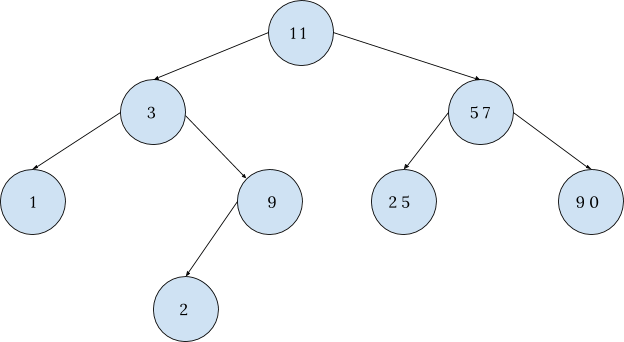The diagram above depicts the situation described as under case 1. Following the steps as per Case 1.

We have the tree after deleting 2 as: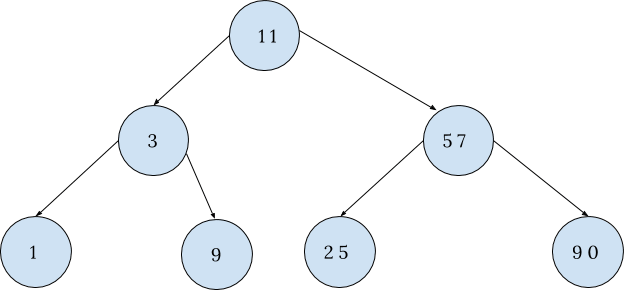1. Traversing

Traversing is the task of visiting all nodes of the tree at least once. The tree is not a linear data structure, hence there are many ways of traversing it. The three most commonly used traversing methods are:

1. In-order
2. Pre-order
3. Post-order

1. In-order traversal: In this traversal mode, one starts visiting with the left child, followed by its parent and then the right child.

Start traversing from the first left child, which in our case is 2. Since 2 is also is a root, we move to its left child. Thus, the first number we traverse is 1, after which we move to its root that is 2 followed by the right child. The right child here is 9, but is a root and also has a left child thus we traverse to the left child 3. Coming back to its root we now visit 9. After this, we visit the root 2 which we've already visited. Thus move to its root 11. After this, we move on to the right sub-tree. The same approach is followed as we did for traversing the left sub-tree.

Thus the final order would be: 1, 2, 3, 9, 11, 13, 17, 25, 57, 90.

2. Pre-Order Traversal:

The order of visitation is as: start from the root, move to left child, then right child. Considering the same tree as in the diagram above, we start the pre-order traversal in the following manner:

In preorder traversal, we follow the order of visiting the root first followed by the left child then the right child. Thus in the diagram given above the root is 11 so first, we visit 11. Now we visit the first left child of 11 that is 2, again we visit the first left child of 2 that is 1.

We did this because 2 is a root and 1 is the left child of 2, and have followed the first two steps of preorder traversal. Now since 2 and 1 are the root and left child, show the next step would be to visit the right child.

The right child of 2 her is 9 but 9 is also a root. so according to pre-order traversal, we have visited the root now we shall visit its left child. The left child of 9 is 3. Thus, we have completed the traversal of the entire left subtree of 11.

Now we shall traverse the right subtree of 11.

Following the same approach, we will traverse the right subtree.

Thus the final order of preorder traversal will be 11, 2, 1, 9, 3, 13, 57, 25, 17, 90.

3. Post-Order Traversal:

The order of visitation is as: start from the left child, move to the right child, and terminate at the root.

Considering the same tree as in the diagram above we start the post order traversal in the following manner:

In post-order traversal, we follow the rule as visit the left child first followed by the right child then the root. Here since 11 is the root we will not visit it but will move to its left child that is 2. That again 2 is a root and it also has a left child which is 1 so first we visit 1, and move to the right child of 2. the right child of 2 is 9 but it also is a root. Again we move to the left child of 9 which is 3. Thus the order of traversal now is 1, 3, 9, 2. Order of visitation is left, left, right and root.

Since we have completed traversing the entire left subtree of the root 11, we move on to the right child of 11 that is 13. In a similar fashion, we visit each and every node of the right subtree of 11 and then, at last, we come to the final root 11.

The final traversal order is 1, 3, 9, 2, 17, 25, 90, 57, 13, 11.

### Pseudocode

Iterative Method:

``````Insert(T, z)
y := nil
x := T.root
while x != nil loop
y := x
if z.key < x.key then
x := x.left
else
x := x.right
end if
end loop

z.parent := y		--x reaches nil, so y is a leaf and becomes parent of z

if y = nil
T.root = z   		-- tree was empty
elsif z.key < y.key
y.left := z
else
y.right := z
end if``````

Recursive Method:

``````Node* Insert(T, data)
If T = nil
return newNode(data)
elsif  T.value < data
T.right := Insert(T.right, data)
else
T.left := Insert(T.left, data)
end

return T
``````

### Implementation:

Following are C, C++, Java and Python implementations of Binary Search Tree (BST).

``````Implementation of BST in C:
#include<stdio.h>
#include<stdlib.h>

struct node              // Structure defined for each node
{
int value;
struct node* left;
struct node* right;
};

struct node* createNode(int data){       //function which initializes a new node
int nodeSize = sizeof(struct node);
struct node* newNode = malloc(nodeSize);
newNode->value = data;
newNode->left = NULL;
newNode->right = NULL;

return newNode;
}

struct node* insertNode(struct node* current, int data)    //inserts a node in BST
{
if (current == NULL)     // if the current reaches NULL, data is to be inserted here
return createNode(data);
if (current->value < data)
current->right = insertNode(current->right, data);
else if (current->value > data)
current->left  = insertNode(current->left, data);

return current;
}

void inOrderTraversal(struct node* current){  //recursive code to print in-order
if(current == NULL)  // reaches end of any leaf and can’t go any deeper in tree
return;

inOrderTraversal(current->left);
printf("%d \n", current->value);
inOrderTraversal(current->right);
}

int main(){
struct node *root;
root  = NULL;
root = insertNode(root, 8);
insertNode(root, 3);
insertNode(root, 1);
insertNode(root, 6);
insertNode(root, 7);
insertNode(root, 10);
insertNode(root, 14);
insertNode(root, 4);

inOrderTraversal(root);
return 0;
}``````
``````Implementation of binary search tree (BST) in C++ :

#include<bits/stdc++.h>
using namespace std;

struct treeNode{
treeNode *left;
treeNode *right;
int value;
} ;
class bst{
treeNode *root;
public:
bst(){
root=NULL;
}
bool isempty() {
if(root==NULL)
return true;
else
return false;
}

void insertNode(int item){
treeNode *p=new treeNode;
treeNode *parent;
p->data=item;
p->left=NULL;
p->right=NULL;
parent=NULL;
if(isempty()){
root=p;
}else{
treeNode *ptr;
ptr=root;
while(ptr!=NULL){
parent=ptr;
if(item>ptr->data)
ptr=ptr->right;
else
ptr=ptr->left;
}
if(item<parent->data){
parent->left=p;
}else{
parent->right=p;
}
}
}

void inOrderTraversal(){
inOrderRec(root);
}

void inOrderRec(treeNode *ptr){
if(ptr!=NULL){
inOrderRec(ptr->left);
cout<<"  "<<ptr->data<<"     ";
inOrderRec(ptr->right);
}
}

void preOrderTraversal(){
preOrderRec(root);
}

void preOrderRec(treeNode *ptr){
if(ptr!=NULL){
cout<<"  "<<ptr->data<<"     ";
preOrderRec(ptr->left);
preOrderRec(ptr->right);
}
}

void postOrderTraversal(){
postOrderRec(root);
}

void postOrderRec(treeNode *ptr){
if(ptr!=NULL){
postOrderRec(ptr->left);
postOrderRec(ptr->right);
cout<<"  "<<ptr->data<<"     ";
}
}
};

int main()
{
bst b;
b.insertNode(52);
b.insertNode(25);
b.insertNode(50);
b.insertNode(15);
b.insertNode(40);
b.insertNode(45);
b.insertNode(20); cout<<"inorder"<<endl;
b.inOrderTraversal();
cout<<endl<<"postorder"<<endl;
b.postOrderTraversal();
cout<<endl<<"preorder"<<endl;
b.postOrderTraversal();

}
``````
``````Implementation of binary search tree in Java:

public class BST {

// Class defining each node with some value and its childs
class TreeNode {
int value;
TreeNode left, right;

public TreeNode(int item) {
value = item;
left = right = null;
}
}

// Root of BST
TreeNode root;

// Constructor
BST() {
root = null;
}

// Wrapper function to call insertRec
void insert(int value) {
root = insertRec(root, value);
}

// Function to insert value in BST recursively
TreeNode insertRec(TreeNode root, int value) {

// returns a new node if it reaches NULL
if (root == null) {
root = new TreeNode(value);
return root;
}

//  Or we can move deeper in the tree
if (value < root.value)
root.left = insertRec(root.left, value);
else if (value > root.value)
root.right = insertRec(root.right, value);

return root;
}

// Wrapper function to call inOrderRec()
void inOrder() {
inOrderRec(root);
}

// Function to print inorder traversal of tree recursively
void inOrderRec(TreeNode root) {
if (root != null) {
inOrderRec(root.left);
System.out.println(root.value);
inOrderRec(root.right);
}
}

// Driver Program to test above functions
public static void main(String[] args) {
BST tree = new BST();

tree.insert(50);
tree.insert(35);
tree.insert(25);
tree.insert(40);
tree.insert(75);
tree.insert(65);
tree.insert(85);

// print inOrder traversal of the BST
tree.inOrder();
}
}
``````
``````Implementation of BST in Python:
# Python program to demonstrate insert operation in binary search tree

#Defining each node containing some value and its children
class TreeNode:
def __init__(self,key):
self.left = None
self.right = None
self.data = key

# Function to insert node in tree recursively
def insertNode(root,node):
if root is None:
root = node
else:
if root.data < node.data:
if root.right is None:
root.right = node
else:
insertNode(root.right, node)
else:
if root.left is None:
root.left = node
else:
insertNode(root.left, node)

# Function to print inorder traversal recursively
def inOrderTraversal(root):
if root:
inOrderTraversal(root.left)
print(root.data)
inOrderTraversal(root.right)

# Creating a new BST with root as 50
r = TreeNode(55)
insertNode(r,TreeNode(35))
insertNode(r,TreeNode(25))
insertNode(r,TreeNode(45))
insertNode(r,TreeNode(75))
insertNode(r,TreeNode(65))
insertNode(r,TreeNode(85))

# Print inoder traversal of the BST
inOrderTraversal(r)
``````

## Serious about Learning Programming ?

Learn this and a lot more with Scaler Academy's industry vetted curriculum which covers Data Structures & Algorithms in depth.
Examples

## Tree Data Structure Problems

Bst traversal
Problem Score Companies Time Status
Valid BST from Preorder 100 60:23
Kth Smallest Element In Tree 300 29:17
2-Sum Binary Tree 400 63:53
BST Iterator 500 41:32
Recover Binary Search Tree 750 65:30
Trie
Problem Score Companies Time Status
Xor Between Two Arrays! 150 47:49
Hotel Reviews 200 74:50
Shortest Unique Prefix 350
69:20
Simple tree ops
Problem Score Companies Time Status
Path to Given Node 200 42:03
Remove Half Nodes 200 46:11
Nodes at Distance K 200 49:41
Balanced Binary Tree 275 30:19
Maximum Edge Removal 300 51:06
2 trees
Problem Score Companies Time Status
Merge two Binary Tree 200 31:02
Symmetric Binary Tree 300 22:00
Identical Binary Trees 300 11:36
Traversal
Problem Score Companies Time Status
Vertical Order traversal of Binary Tree 200 74:16
Diagonal Traversal 200 47:19
Inorder Traversal 350 32:00
Preorder Traversal 350 15:26
Postorder Traversal 350 31:28
Level order
Root to leaf
Problem Score Companies Time Status
Burn a Tree 200 58:35
Max Depth of Binary Tree 350 6:57
Sum Root to Leaf Numbers 350
45:33
Path Sum 350 27:35
Min Depth of Binary Tree 350 17:20
Root to Leaf Paths With Sum 350 41:13
Inplace change
Problem Score Companies Time Status
Invert the Binary Tree 300
12:46
Tree search
Problem Score Companies Time Status
Least Common Ancestor 450 57:33
Problem Score Companies Time Status
Flatten Binary Tree to Linked List 500 43:45
Segment tree
Problem Score Companies Time Status
Order of People Heights 700
75:58Free Mock Assessment
Help us know you better for the best experience
Current Employer *
Enter company name